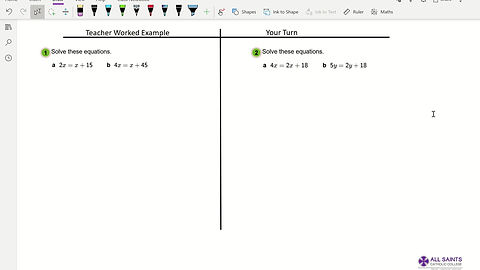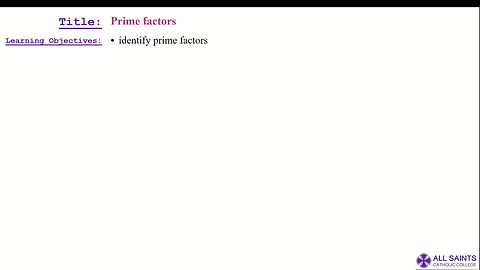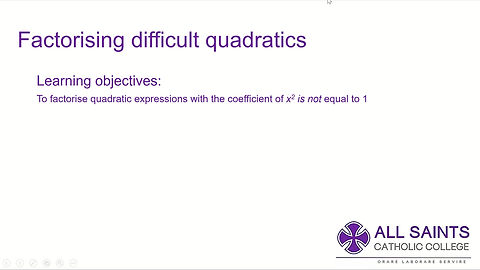## Yr 10 MathsFoundation Tier - Algebra 1Foundation Tier - Algebra 2Foundation Tier - Algebra 3Foundation Tier - Algebra 4Foundation Tier - ExpectationFoundation Tier - Volume Of A CylinderFoundation Tier - Surface Area Of A Triangular PrismFoundation Tier - Solving Equations Using Balancing MethodsFoundation Tier - Solving Fractional EquationsFoundation Tier - Solving Equations With A Variable X On Both SidesFoundation Tier - Prime Factor DecompositionFoundation Tier - Sum Of Interior AnglesFoundation Tier - Exterior and Interior Angles Of PolygonsHigher Tier - Pythagoras TheoremHigher Tier - SOH CAH TOAHigher Tier - Missing Lengths And Areas in Similar ShapesHigher Tier - Volume Of Similar ShapesHigher Tier - Standard Form Calculator MethodHigher Tier - Solving Equations With BracketsHigher Tier - Solving Equations - Expanding BracketsHigher Tier - Solving Simultaneous Linear EquationsHigher Tier - Multiplying & Dividing Powers Using Index LawsHigher Tier - Solving Quadratic Equations By FactorisationHigher Tier - Solving Difficult Quadratic Equations By FactorisationHigher Tier - Factorising Difficult QuadraticsHigher Tier - The Quadratic FormulaHigher Tier - Quadratic Equations Revision